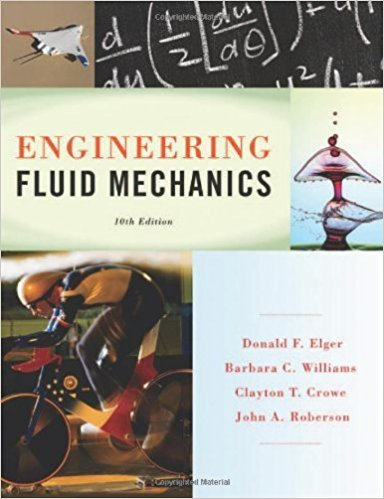> > > > Problem 2.57

# Which of the following is the formula for the gage pressure within a very small## Problem 2.57 Chapter 2

Engineering Fluid Mechanics | 10th Edition

• 2901 Step-by-step solutions solved by professors and subject experts
• Get 24/7 help from StudySoup virtual teaching assistantsEngineering Fluid Mechanics | 10th Edition

4 5 0 270 Reviews
14
3
Problem 2.57

Which of the following is the formula for the gage pressure within a very small spherical droplet of water: (a) p = u /d, (b) p = 4u/d, or (c) p = 8u/d?

Step-by-Step Solution:
Step 1 of 3

Thomas 1 Trigonometry Example: Pythagorean Theorem: Scalars & Vectors Scalar quantities are just a number 1. Examples: a. Temperature b. Height c. Mass 2. Note that scaler quantities exclude physical quantities Vector Quantities 1. Vectors are described by magnitude and direction arrows. a....

Step 2 of 3

Step 3 of 3

##### ISBN: 9781118164297

Unlock Textbook Solution

Which of the following is the formula for the gage pressure within a very small

×
Get Full Access to Engineering Fluid Mechanics - 10 Edition - Chapter 2 - Problem 2.57

Get Full Access to Engineering Fluid Mechanics - 10 Edition - Chapter 2 - Problem 2.57

I don't want to reset my password

Need help? Contact support

Need an Account? Is not associated with an account
We're here to help